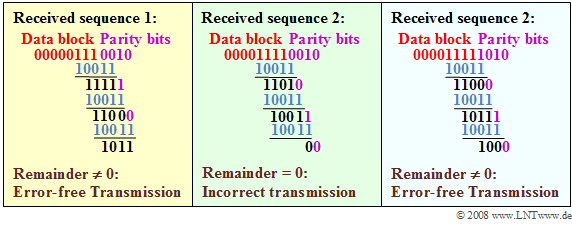# Exercise 1.6: Cyclic Redundancy Check

The synchronization happens at the primary multiplex connection in each case in synchronization channel  "$0$"  of each frame:

1. For odd time frames  (number 1, 3, ... , 15)  this transmits the so-called  "frame password"  with the fixed bit pattern  $\rm X001\hspace{0.05cm} 1011$.
2. Each even frame  (with number 2, 4, ... , 16)  on the other hand contains the  "message word"  $\rm X1DN\hspace{0.05cm}YYYY$.
3. Error messages are signaled via the  $\rm D$  bit and the   $\rm N$  bit.  The four  $\rm Y$  bits are reserved for service functions.

The  $\rm X$  bit is obtained in each case by the  "CRC4 method",  the implementation of which is shown in the diagram:

• From eight input bits each - in the entire exercise the bit sequence  "$\rm 1011\hspace{0.05cm} 0110$"  is assumed for this purpose - the four check bits  $\rm CRC3$, ... , $\rm CRC0$  are obtained by modulo-2 additions and shifts,  which are added to the input word in this order.
• Before the first bit is shifted into the register,  all registers are filled with zeros:
$${\rm CRC3 = CRC2 =CRC1 =CRC0 = 0}\hspace{0.05cm}.$$
• After eight shift clocks,  the four registers  $\rm CRC3$, ... , $\rm CRC0$  contains the CRC4 checksum.

The taps of the shift register are  $g_{0} = 1, \ g_{1} = 1, \ g_{2} = 0, \ g_{3} = 0$  and  $g_{4} = 1$.

• The corresponding generator polynomial is:
$$G(D) = D^4 + D +1 \hspace{0.05cm}.$$
• The CRC4 checksum on the transmission side is also obtained as the   "remainder"   of the polynomial division
$$(D^{11} +D^{9} +D^{8}+D^{6}+D^{5})/G(D) \hspace{0.05cm}.$$
• The divisor polynomial results from the input sequence and four appended zeros:  "$\rm 1011\hspace{0.09cm} 0110\hspace{0.09cm} 0000$".

The CRC4 check at the receiver according to subtask  (4)  can also be represented by a polynomial division.  It can be implemented by a shift register structure in a similar way as the CRC4 checksum is obtained at the transmitting end.

Notes:

### Questions

1

Which result  $E(D)$  and which remainder  $R(D)$  results from the polynomial division $(D^{11} + D^{9} + D^{8} + D^{6} + D^{5}) : (D^{4} + D + 1)$ ?

 $E(D) = D^{5} + D^{3} + 1, \hspace{2.13cm}R(D) = D^{3} + D$, $E(D) = D^{7} + D^{5} + D^{3} + 1, \hspace{1cm}R(D) = D^{3} + D + 1$, $E(D) = D^{7} + D^{5} + D^{3} + 1, \hspace{1cm}R(D) = 0$.

2

What is the CRC checksum in the present case?

 $\rm CRC0 \ = \$ $\rm CRC1 \ = \$ $\rm CRC2 \ = \$ $\rm CRC3 \ = \$

3

The following bit sequences arrive at the receiver,  eight information bits each plus  $\text{ (CRC3, CRC2, CRC1,CRC0)}$.
What bit sequences indicate that there is no bit error?

 $1011 \hspace{0.1cm}0010\hspace{0.08cm} 1011$, $1011 \hspace{0.1cm}0110 \hspace{0.08cm}1011$, $1011 \hspace{0.1cm}0110\hspace{0.08cm} 1001$.

4

Which of the following received bit sequences were falsified during transmission?

 $0000 \hspace{0.1cm}0111 \hspace{0.1cm}0010$, $0000 \hspace{0.1cm}1111\hspace{0.1cm} 0010$, $0000\hspace{0.1cm} 1111\hspace{0.1cm} 1010$.

### Solution

#### Solution

(1)  The  Solution 2  is correct:

• Due to the largest numerator exponent  $(D^{11})$  and the highest denominator exponent  $(D^{4})$,  the first suggestion  $E(D) = D^{5} + D^{3} + 1$  can be excluded as the result   ⇒   $E(D) = D^{7} + D^{5} + D^{3} + 1$.
• Modulo-2 multiplication of  $E(D)$  by the generator polynomial  $G(D) = D^{4} + D + 1$  yields:
$$E(D) \cdot G(D) \ = \ (D^7+ D^5+D^3+1)\cdot (D^4+ D+1) \ = D^{11}+D^8+D^7+D^9+D^6+D^5+D^7+D^4+D^3+D^4+ D+1 \hspace{0.05cm}.$$
• It must be taken into account here that for modulo-2 calculations $D^{4} + D^{4} = 0$. This results in the following remainder:
$$R(D) = D^{11}+D^9+D^8+D^6+D^5- E(D) \cdot G(D) = D^3+D+1 \hspace{0.05cm}.$$

(2)  From the result of subtask  (1)  follows:

$${\rm CRC0 = 1},\hspace{0.2cm}{\rm CRC1 = 1},\hspace{0.2cm}{\rm CRC2 = 0},\hspace{0.2cm}{\rm CRC3 = 1}\hspace{0.05cm}.$$
• The table shows a second way of solution:
• It contains the register assignments of the given circuit at times  $0$, ... , $8$.

(3)  Only  solution 2  is correct:

• The receiver divides the polynomial  $P(D)$  of the received sequence by the generator polynomial  $G(D)$.
• If this modulo-2 division returns the remainder  $R(D) = 0$,  then all  $12$  bits were transmitted correctly.
• This is true for the second solution,  as a comparison with subtasks  (1)  and  (2)  shows.  It is valid without remainder:
$$(D^{11}+D^9+D^8+D^6+D^5+D^3+D+1) : (D^4+ D+1)= D^7+D^5+D^3+1 \hspace{0.05cm}.$$
• In solution 1 the 6th information bit was falsified,  in solution 3 the CRC1 bit.

(4)  Solutions 1 and 3 are correct:Polynomial division of the three received sequences
• The diagram illustrates the modulo-2 divisions for the given received sequences in simplified form  (with zeros and ones).
• You can see that only for sequence 2 the division is possible without remainder.
• In written form, is possible the polynomial divisions are:

$$\ (1) \ \hspace{0.2cm}(D^6+D^5+D^4+1) : (D^4+ D+1)$$

$$\hspace{0.3cm}\Rightarrow \hspace{0.3cm}\text{remainder:}\hspace{0.15cm}D^3+ D+1\hspace{0.05cm},$$

$$\ (2) \ \hspace{0.2cm}(D^7+D^6+D^5+D^4+1) : (D^4+ D+1)$$

$$\hspace{0.3cm}\Rightarrow \hspace{0.3cm}\text{remainder:}\hspace{0.15cm}0\hspace{0.05cm},$$

$$\ (3) \ \hspace{0.2cm}(D^7+D^6+D^5+D^4+D^3+1) : (D^4+ D+1)$$

$$\hspace{0.3cm}\Rightarrow \hspace{0.3cm}\text{remainder:}\hspace{0.15cm}D^3\hspace{0.05cm}.$$